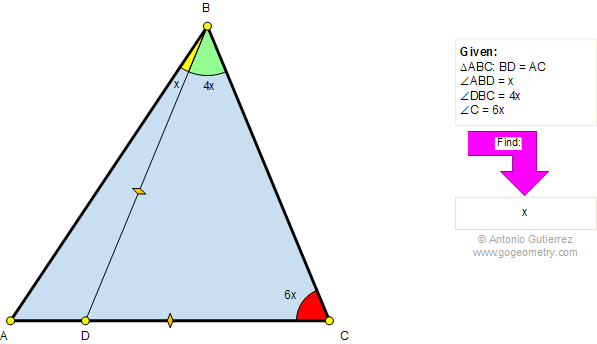Problem 516. Triangle, Cevian, Congruence, Angles Level: High School, College, SAT Prep. The figure shows a triangle ABC and a cevian BD equal to AC. If angle ABD = x, angle DBC = 4x, and angle C = 6x, find x. A cevian is a line segment which joins a vertex of a triangle with a point on the opposite side (or its extension).Poster and typography of problem 516.Recent Additions